Chemistry

## Quiz 2 : Structural Analysis of a DipeptideLooking for Biochemistry Homework Help?# Quiz 2 : Structural Analysis of a Dipeptide

Substitute the above obtained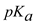value in equation (1),So, 0.5 M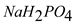=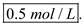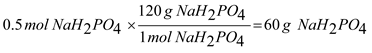Thus, the number of grams of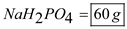. 0.308 M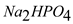= 0.308 mol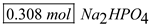/L, so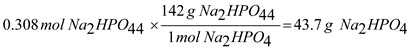Thus, the number of grams of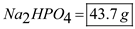.

In this problem, we are asked to prepare a liter of 0.5 M sodium phosphate buffer, pH 7.0, using only monobasic sodium phosphate (NaH2 PO 4 ), a sodium hydroxide (NaOH) solution, and a pH meter. By using the monobasic phosphate, we can measure out directly 0.5 moles of this material and dissolve in around 900mL of water (we need a liter total, but we want to make sure the pH is correct before we fill the solution to completion). After the phosphate is dissolved in the water, we use our pH meter to determine the pH, and then adjust drop-wise with NaOH until the pH is 7.0; the salt is acidic, so the pH will initially be below 7.0. Once the pH is in range, we fill the vessel to one liter.

In this problem, we are asked to explain the procedures to prepare a 0.1M glycine buffer at pH 10.0. We will use the Henderson-Hasselbalch equation to calculate the moles of isoelectric glycine and sodium glycinate we will need. The Henderson-Hasselbalch equation is as follows: pH = -log (Ka) + log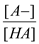Where: pH the pH of the solution Ka the acid dissociation constant for HA [A-] concentration of the anion of the acid [HA] concentration of the acid The acid in this buffer is the glycine, and the anion of this combination is sodium glycinate. The Ka for isoelectric glycine ion is 2.51x10 -10. We calculate the ratio of our anion to the acid as follows: pH = -log (Ka) + logWe also know that the [A-] + [HA] = 0.1M. We can say that [HA] = 0.1 - [A-].Thus, [A-] equals 0.07 moles, and because the two must add up to 0.1M, the [HA] must be 0.03 moles. This would be added into a 1L volumetric flask and then filled to the mark.

There is no answer for this question

There is no answer for this question